# The Limitless Vertigo of Cantor’s Infinite

Mathematics is often presented as the one human endeavour whose claims are indisputable. Yet, when, in the late 19th century, the giant Georg Cantor would dare to theorize mathematically about the infinite, not only would he initiate everlasting debates with the Church and philosophers, but he’d also undergo brutal objections by fellow mathematicians.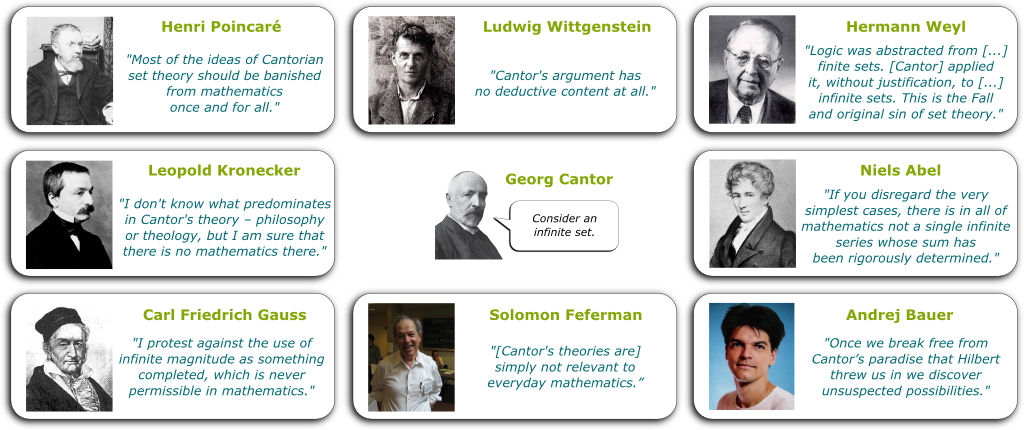Cantor was particularly maltreated by Kronecker, who would describe him as a “scientific charlatan“, a “renegade” and a “corrupter of youth.” In fact, in his (sane) lifetime, Cantor would find hardly any supporter. Instead, the greatest mathematicians of his time would look down on him. They wouldn’t hesitate to bring him down. The doors of of the greatest mathematical institutes would be closed to him. Cantor was unwelcome. Alone against the whole mathematical community, he would feel isolated to the point of doubting his own ideas. In the end, it is not so surprising that the great genius eventually went a bit crazy.

You’re talking as though he was right against the mathematical community? Was he? And if he were right, could it be that absolutely no one believed him?

Cantor did not have many fans. But he did have a few. In particular, fortunately for Cantor, one of the fans was the most charismatic mathematician of that time, the great David Hilbert. Hilbert viewed Cantor’s achievements as of the greatest order.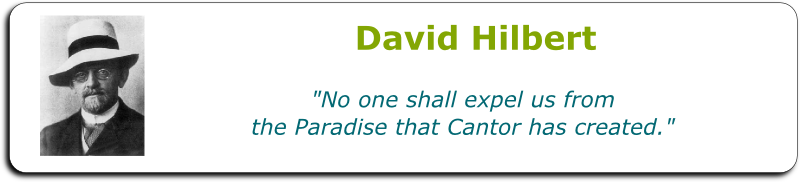At last, Cantor’s theory that was being a rejected by the whole mathematical community would be brandished by its leader.

Wow! That sounds so unlikely! But how does the mathematical community now feels about Cantor’s theory?

Today, some serious mathematicians like Norman Wildberger still refute Cantor, while others like Andrej Bauer invite us to disregard Cantor’s theory (at least every now and then). But overall, the mathematical community celebrates it as one of mankind’s greatest achievements. Cantor’s ideas are now taught in all the universities in the world as some of the most beautiful truths in mathematicss.

This is so intriguing! So what was Cantor’s theory?

Hehe… Among other things, Cantor would prove that there were different infinities, with some infinities that are bigger than others…

## Hilbert’s Hotel

A quick way to get a feeling of the vertigo of Cantor’s theory of the infinite is to follow the footsteps of David Hilbert. To give an insight into Cantor’s theory, Hilbert proposed a clever thought experiment. Imagine a hotel with an infinite number of rooms.

What do you mean?

I mean a hotel with a room numbered 1, a room numbered 2, a room numbered 3… and so on to infinity.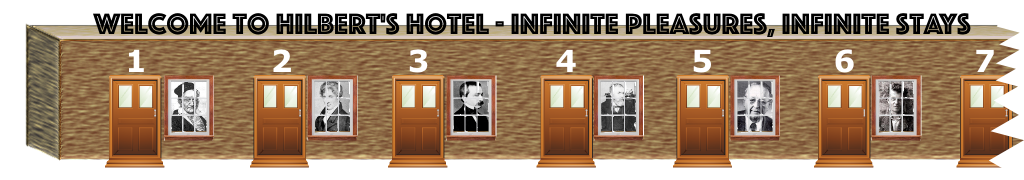OK…

Now, imagine that the hotel is full, and that a newcomer arrives at the desk office. Could the hotel manager, say Hilbert himself, find a room for the newcomer?

Obviously not! The hotel is full!

Are you sure? If the hotel was finite, your answer would be perfectly right. But don’t forget. Hilbert’s hotel is infinite.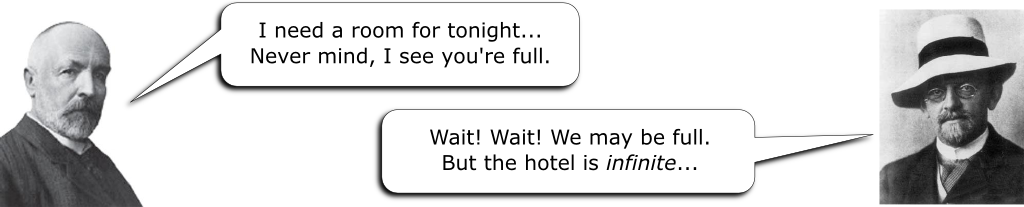Hummm… I don’t see how to free a room…

Take your time. You can figure this one out!

Hummm…

Okay, here’s the trick. Hilbert could ask every guest to move to the room next to his, hence shifting all the guests by one unit. Every guest would still be having a room. But amazingly, they would also have freed the first room! There’s room for the newcomer!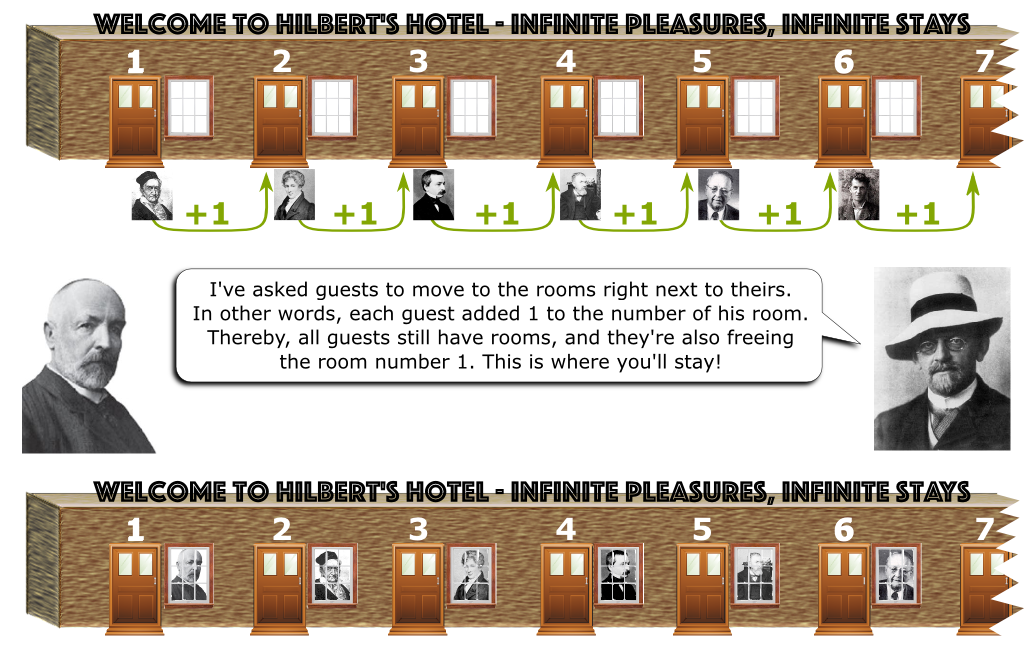Wow! That is so counter-intuitive!

I know! Think about what has just happened. We’ve shown that an infinite hotel can always accommodate a newcomer, even when it is full! Mathematically, by adding one to all guests’ rooms, we’ve also shown that there are as many whole numbers as there are whole numbers larger or equal to 2! If you’ve never heard about that, it should blow your mind!

It does!

And yet, we’re merely at the beginning of our journey in the world of the infinites! Let’s go further. Let’s now imagine that there is an infinite number of newcomers. Could Hilbert accommodate them all?

Hummm… Well, he could repeat this operation indefinitely, right?

I guess… But that would take an infinite amount of time. Can Hilbert free an infinite number of room at once?

Hummm… By moving the first guest to room number 2, we’re freeing one room.

Yes. That’s what we’ve done.

But now, let’s also move the second guest to room number 4, so that we free room number 3.

You’re onto something!

Then, the third guest to room number 6 to free room number 5.

Do you see the pattern?

I do! We’ll send the $n$th guest to room number $2 \times n$… We’re doubling the guests’ room numbers!

Awesome! Thereby, we’re freeing all the odd-numbered rooms!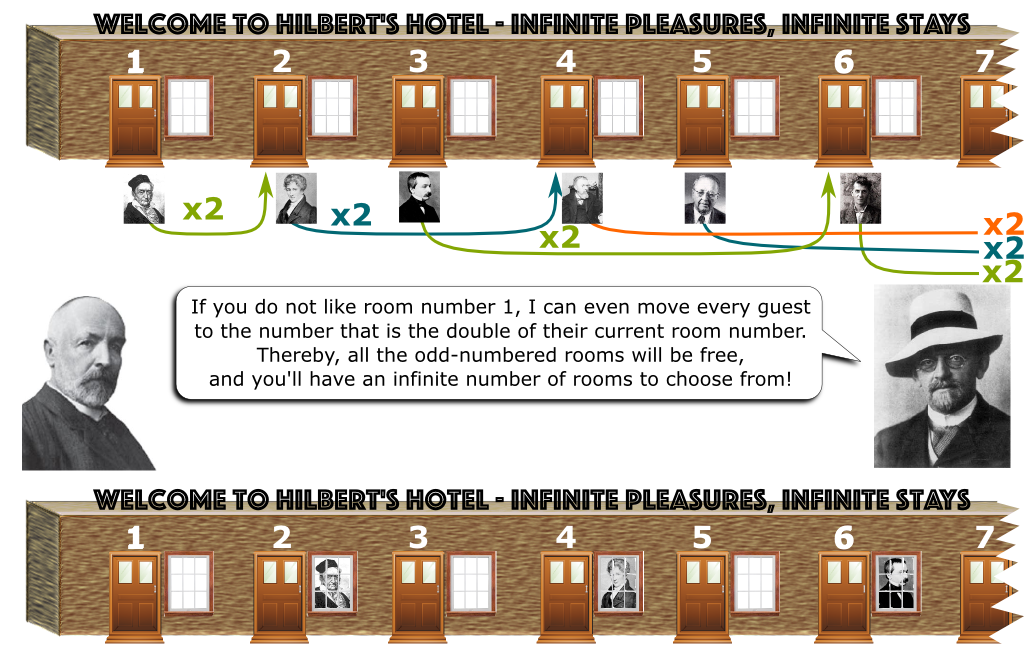Amazingly, we’ve freed an infinite number of room at once! Through this doubling rule, we’ve shown that we can fit the set of whole numbers into the set of even numbers. This is astounding! It’s what’s brilliantly explain in this video by the Open University Youtube channel:

You haven’t answered my question though. Can Hilbert fit an infinite number of newcomers in his full hotel?

Well, obviously, yes, since we’ve freed an infinite number of rooms!

Obviously?

Sure! You take a list of all the newcomers. The first of the list will go to room number 1, the second to room number 3, the third to room number 5… and so on.

Don’t you see where you got it wrong?

Did I get something wrong?

You did. You assumed that the newcomers could be listed!

Can’t all sets be listed?

As astounding as it sounds, Cantor would prove that no. Some sets aren’t listable.

The classical word is rather “countable”. But as James Grime brilliantly points it out on Numberphile, “countable” is a very poor terminology. Infinite countable sets cannot be counted, precisely because they are infinite. Instead, we should talk about listable and unlistable sets.

## Listable Infinity

Let’s start with listable infinities. The most obviously listable of these infinities is evidently the whole numbers. Let me write that as a theorem with a proof.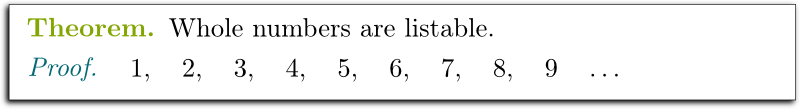That wasn’t hard, was it?

Nope. I got it!

Now, what about positive and negative integers?

Hummm…

What could be the first element of this list of integers? What could the second? The third? The one after that?

How about the list: 0, 1, -1, 2, -2, 3, -3,… and so on?

Very good! Let me present that as a beautiful theorem: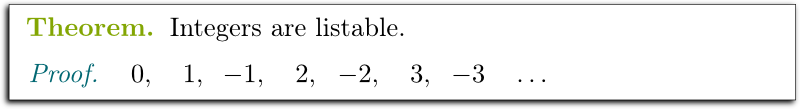Now that we have seen two examples of infinite listable sets, I can get to Cantor’s big idea. As I said in the introduction, Cantor would prove that some infinities are bigger than other infinities. But what could he mean by that?

I guess it means that some infinities have more elements than others…

But what does that mean? By far, Cantor’s greatest idea was to give a meaning to the “size” of a (possibly infinite) set. To understand Cantor’s insight, let’s play a game. In the following picture, are there more apples than bananas?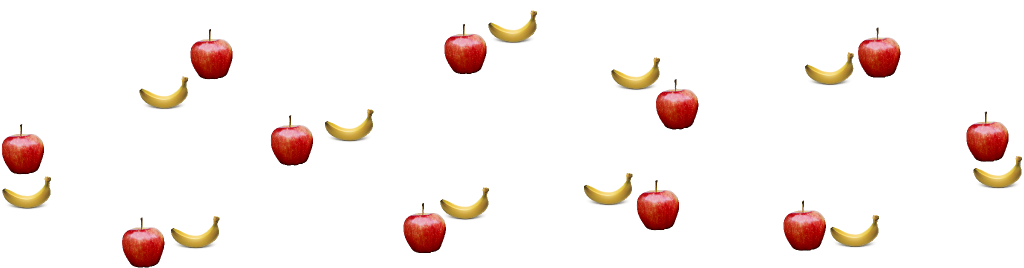Easy! There are as many apples as bananas?

How do you know? Did you count them? How many of each are there?

I don’t know… I actually don’t know how I know what I know! But I know I didn’t count them.

I know. But then, how do you know that there are as many apples as bananas?

Hummm… It’s clear, isn’t it?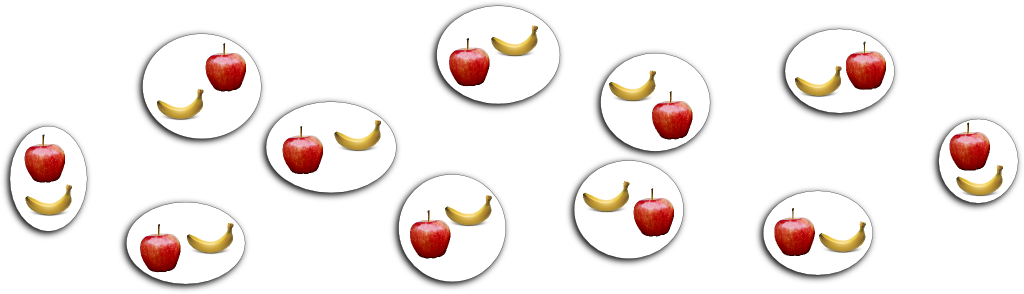Do you see it now?

I do! I’ve managed to pair them up!

Now this is a brilliant insight. To guarantee the fact that two sets have the same size, you don’t need to count their elements. You just need to pair up their elements. In fact, this is how Georg Cantor decided to define what it meant for two sets to have the same size. And that, my friends, is Cantor’s greatest idea!

Really? His greatest idea?

As we’ve seen, it’s obviously a good definition when sets are finite. But interestingly, it can be applied to infinite sets too! Without this insight, there would be no Cantorian theory, no set theory, no basic foundational mathematics… we’d be back to the dark ages! OK, I might be exaggerating a little bit, but, seriously, Cantor’s definition is undeniably a major breakthrough that would inspire later mathematicians to take on the extremely fruitful abstraction of mathematical concepts.

A pairing of all elements of two sets is called a bijection. Formally, two sets have the same size when there is exists a bijection between them.
OK… But what does that have to do with the listability of sets?

Suppose you can list an infinite set of apples and an infinite set of bananas. Do you see a way to pair up apples and bananas?

Yes! I can pair up the first apple with the first banana, the second apple with the second banana… and so on!

Exactly! In fact, we have the following wonderful theorem.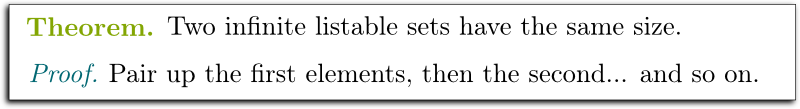There’s more. A set $A$ has the same size as an infinite listable set $L$ if and only if $A$ is itself infinite listable. Indeed, if $A$ is in bijection with $L$, then we can list its elements, by determining the $n$th element of $A$ as the one that’s paired with the $n$th element of $L$.

By combining the three theorems we have seen, we can now assert one troubling fact. There are as many whole numbers as integers!

Wow!

Now, let’s move on to a bigger test. You do know about rational numbers, don’t you?

There are like 2/3, 5/2… and so on, right?

Exactly! Can you list the rational numbers?

Well, there seems to be a lot of rational numbers…

I know! As a matter of fact, between any two rational numbers, you can always find another rational numbers. In fact, you can find infinitely many rational numbers between any two rational numbers. So there seems to be a lot of rationals.

So I guess that there are more rationals than whole numbers…

Are you sure?

Well, no… What’s the answer? Can they be listed?

Hehe… They can! To do so, let’s first represent them in an array accordingly to the numerators and denominators: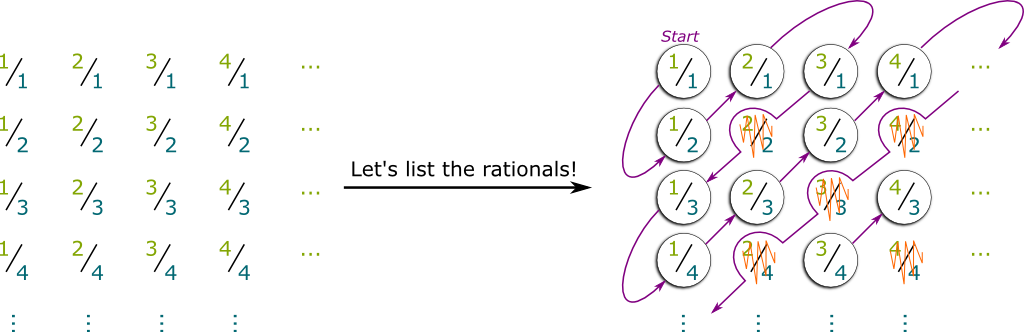Now, here’s the big idea. We can list the rationals by going through the down-left-to-up-right diagonals, from the inside to the outside, as we jump over the rationals we’ve already gone through. Thereby, we have a list of all positive rationals.

Of course, what I asked for is a list of all rationals. But, based on how we listed integers and how we listed positive rationals, I’ll let you guess by yourself how all rationals could be listed.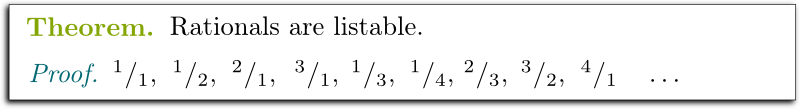Now, what I’ve presented here is the classical proof that rationals are listable. But I want to tell you about a more elegant proof. In fact, you know what? I’ll let the great Matt Parker tell it to you, on Numberphile:

As an exercise, try to list the set of $n$-tuples of rationals, and the set of polynomials with rational coefficients (that is, of the form $a_n X^n + a_{n-1} X^{n-1} + \ldots + a_1 X + a_0$).

## Unlistable Infinity

At this point, you might think that all infinities are listable…

Well no. I’ve read the title of this section!

Okay. Fair enough. So then, let’s talk about unlistable infinities. Can you think of an infinite set so big that it cannot be listed?

Hummm…

Let me give it to you! After all, it’s a very, very difficult question. I claim that the decimal numbers — mathematically badly named the “real” numbers — cannot be listed.

Wait what are these real numbers?

They are numbers with an infinite number of decimals. You know? Like $e \approx 2.71828182846…$ or $\tau \approx 6.28318530718…$

Or you might have heard of the impostor $\pi = \tau / 2 \approx 3.14159…$ This number $\pi$ is an impostor, because $\pi$ is wrong!
And you’re saying that these real numbers can’t be listed?

That’s what I’m saying. Any list of the reals would miss out on many of the reals.

Why?

The proof of it is one of the most beautiful proof of mathematics! As you’ve guessed, Cantor is the one who came up with this. His proof is a classic example of a proof by contradiction. Suppose there is a list of reals. Cantor will find something wrong about this list. Namely, he will construct a real number that is not in the list.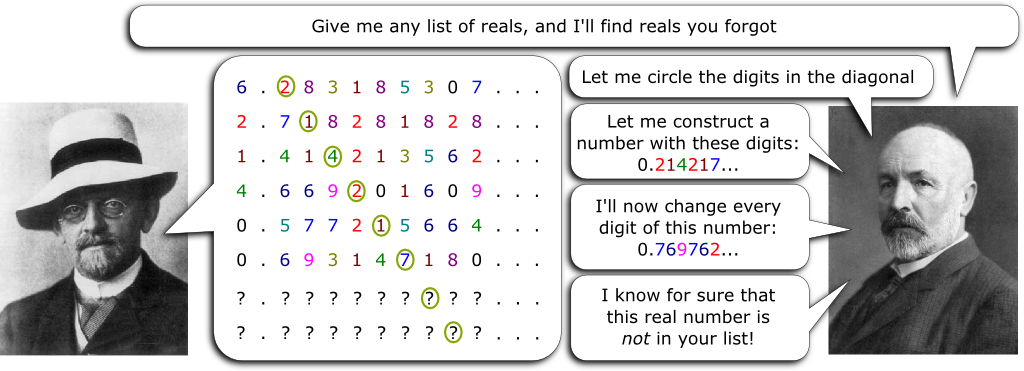To determine a real number that’s not in the list, Cantor first constructed a real number whose $n^{th}$ decimal digit is the $n^{th}$ decimal digit of the $n^{th}$ number of the list. Then, he made up another real number by changing all the digits. This is the number that Cantor claims not to be in the list.

How do you know?

Could Cantor’s number be the $n^{th}$ element of the list?

Hummm… I don’t know.

If it were, then all the digits of Cantor’s number would be the same as the digits of the $n^{th}$ element of the list. Yet, we know by construction that Cantor’s number’s $n^{th}$ decimal digit is not the $n^{th}$ decimal digit of the $n^{th}$ number of the list!

I see! Therefore, their $n^{th}$ decimal digits differ, and, thus, they cannot be the same! That’s brilliant!

I know! Let’s listen to Henry Reich who summed up this wonderful piece of mathematics on Minute Physics:

To be a bit more rigorous, this is not a sufficient argument. Some real numbers can be written in two different ways. For instance, 0.9999999…=1. But if you avoid switching 0s into 9s and vice-versa, and instead switch them into any number between 1 and 8, then you’ll be fine with Cantor’s proof.

I can finally state this as a theorem: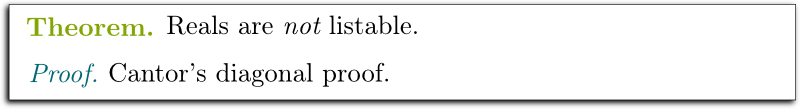In effect, this says that the infinite of real numbers is bigger than the listable infinite!

Wow! And are there still bigger infinities?

There are!

The construction of bigger infinities is such a beautiful piece of mathematics that I can’t resist including it in this article. It is a bit more technical than the rest of the article though, so feel free to jump ahead if you’re struggling with the abstractness of the following paragraphs.

For any infinite set $E$ (like for instance, the set of real numbers), Cantor devised a clever method to construct a bigger infinity $\mathcal P(E)$, called the power set.

How did he do that?

He defined $\mathcal P(E)$ as the set of subsets of $E$.

Wait… What’s a subset?

A subset of $E$ is a set that contains some but not necessarily all elements of $E$. For instance, if $E$ is a set containing an apple and a banana, $E$ has four subsets. One contains both the apple and the banana, another contains only the apple, another contains only the banana, and the last subset contains nothing. More generally, when $E$ has two elements, $\mathcal P(E)$ has four elements.

Hummm… That’s hard to visualize.

Here’s an equivalent perhaps simpler way to visualize the power set of $E$. A subset $E$ can be determined by saying for all elements $e$ of $E$ whether $e$ is in or out the subset. So, back to our apple-and-banana example, a subset is determined by whether the apple is in or out, and whether the banana is in or out. Since there are two possibilities for each element of $E$, there are globally $2^E$ possibilities. That’s why the power set $\mathcal P(E)$ is sometimes denoted $\mathcal P(E) = 2^E$.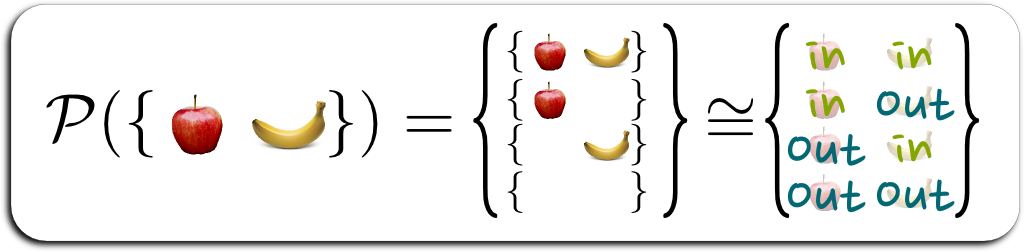More rigorously, we have a canonical bijection $f : \{ in, out \}^E \rightarrow \mathcal P(E)$, which inputs a function $g : E \rightarrow \{ in, out \}$ and outputs the subset $f(g)$ that contains an element $e \in E$ if and only if $g(e) = in$.

Finally, I can state Cantor’s theorem. Cantor proved that the power set $\mathcal P(E)$ is always bigger than the set $E$. In other words, you cannot pair up elements of $E$ and of $\mathcal P(E)$.

Hummm… Why?

The idea of the proof is essentially the same as the proof that reals are not listable. To get the intuition of the proof, let me list elements of $E$ anyways, assume that they are paired with subsets of $E$ and write whether the subset they are paired with leave elements of $E$ in or out…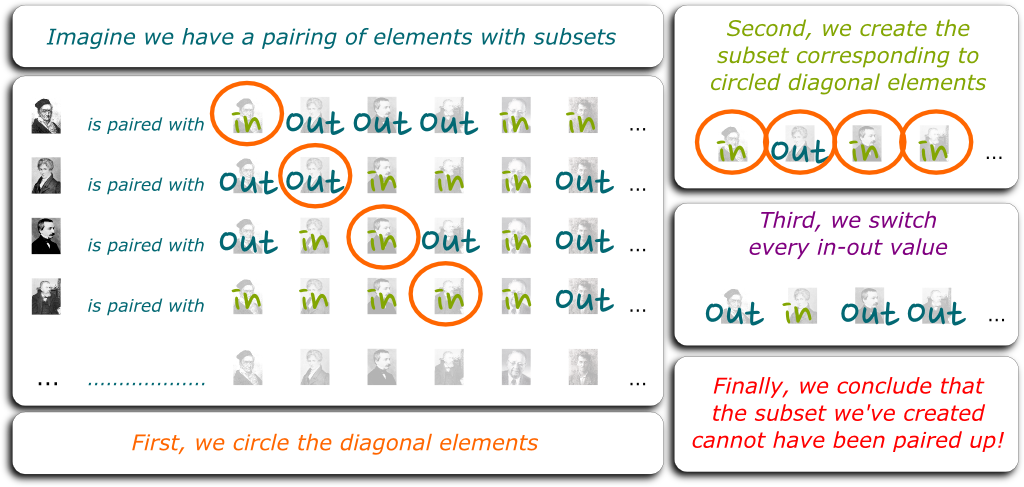Next, like before, we are going to make up a first subset using diagonal terms, and then another subset by switching every in-or-out value. Finally, we obtain a set that could not have been paired up, because of the diagonal argument!

Formally, assume that $f : E \rightarrow \mathcal P(E)$ is a bijection. Let $F = \{ e \in E \; | \; e \notin f(e) \}$ the subset of $E$ that switches the values of the diagonal terms (if $e$ was in $f(e)$, then $e$ is not in $F$, and if $e$ was not in $f(e)$, then $e$ is in $F$). Then, $F$ cannot have been some $f(e)$. Indeed, if $e$ is in $f(e)$, then $e$ is not in $F$. But if $e$ is not in $f(e)$, then it is in $F$. Either way, $F$ and $f(e)$ do not agree on whether they include $e$, thus they cannot be the same subsets. Therefore, we have a contradiction, as $F$ has not been paired up by $f$ with any element $e \in E$.

Do you realize what this means? It means that the power set $\mathcal P(\mathbb R)$ of the set of real numbers is strictly bigger than the set $\mathbb R$ of real numbers. More generally, there is no biggest infinity. Just take the power set of an infinite set to make up a bigger one!

On other note, I’ll let you prove as an exercise that the power set of an infinite listable set has the same size as the set of real numbers.

## The Continuum Hypothesis

Many of Cantor’s proofs are the kinds of enlightening beautiful insights that every mathematician wishes he could stumble upon by himself. But there’s one problem that defied even the great Cantor.

What’s that problem?

Hilbert found that problem so important that he put it at the top of his list of problems to be solved, when he presented a list of 23 open problems in his legendary 1900 talk. These problems would be guiding mathematicians all along the 20th century, and, surely enough, they would yield deep insights into the nature of mathematics. In fact, the one that allowed for the greatest insights might well be the first of these problems, the problem that Cantor could not solve.

Again… What’s that problem?

It is called the continuum hypothesis. It asserts that the infinite of the reals is the smallest infinite bigger than the listable infinite. Cantor and Hilbert could not prove it.

The listable infinite is commonly denoted $\aleph_0$. Its power set has the same size as the real. This size is thus denoted $2^{\aleph_0}$. Meanwhile the smallest infinite bigger than $\aleph_0$ is called $\aleph_1$ (whose existence is guaranteed by the highly questionable axiom of choice). The continuum hypothesis then asks whether $2^{\aleph_0} = \aleph_1$.
Has this problem been solved?

To this day, no one has been able to prove nor disprove the continuum hypothesis.

So, it’s still an open problem, isn’t it?

Actually, the problem is considered solved!

How come? What does that mean?

It’s one of the most dramatic result in the History of mathematics! In 1940, Kurt Gödel would prove that you couldn’t prove the existence of an infinite in-between the infinites of the whole numbers and the reals. In other words, according to Gödel, the continuum hypothesis has no disproof.

Doesn’t that solve the problem? Doesn’t it mean that the continuum hypothesis is true?

Read carefully. Gödel didn’t prove that the continuum hypothesis is true. He proved that you couldn’t prove that it was false.

Hummm… I’m not sure I understand. So, he didn’t solve the problem, did he?

He did not. But in 1963, Paul Cohen would complete Gödel’s answer in the most stunning possible way. He would prove that there is no proof that the continuum hypothesis is true!

Wait… What?

Astoundingly, Gödel and Cohen proved that no one will ever figure out the continuum hypothesis. It cannot be proved, nor can it be disproved!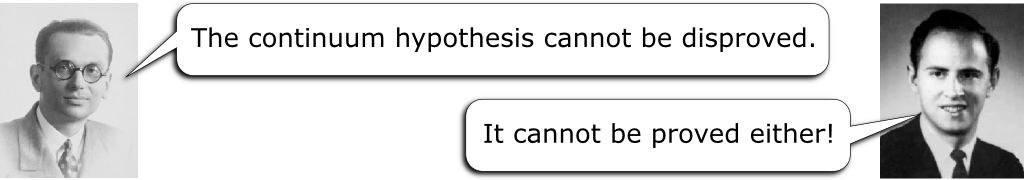Wow! That’s… Is that even possible?

This sort of unprovability had been predicted decades earlier by Kurt Gödel himself, in 1931. It’s called the (first) incompleteness theorem, which asserts that in all mathematical systems, you will always stumble upon assertions that can never be proved nor disproved. But this was a theoretical prediction that didn’t seem to come up in practice. To everyone’s surprise, it did. And not to any problem. It popped up for Hilbert’s most prestigious problem, the continuum hypothesis!

I’m butchering Gödel’s incompleteness theorem here and I’m sorry for that. But it is roughly what it asserts.
So what can be done then?

This means that you can assume that the continuum hypothesis is true, and do one kind of mathematics. But you can just as well assume that it is false, and do another kind of mathematics. These two alternatives would yield two different mathematical universes.

So there’s like… a mathematical multiverse?

Exactly! Especially nowadays, many, many different universes of mathematics have been unveiled. As Andrej Bauer argues, too often though, mathematicians are stuck in their own little universe called ZFC (well, it’s actually not so small since it contains more infinites than any infinite…). ZFC is nothing less than the universe Cantor was exploring, even though he himself could not formalize it. It is also the universe that is brutally taught to students as the only evident mathematical universe one can (or should) work with.

But there are, in fact, other universes…

Yes indeed! Granted, ZFC is Historically the universe mathematicians have mostly worked with. But, as Bauer argues, it’s definitely not the only interesting universe. And the cool thing about the mathematical multiverse is that it is physically possible to explore it — physics never was and never will be a limitation to mathematics! Thus, Bauer invites mathematicians to take a trip towards other universes and discover some of the unusual, but probably useful, properties of the mathematical objects they’ll encounter!